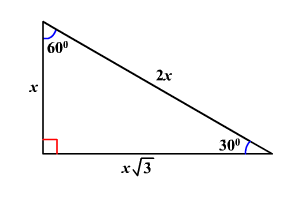# 30°-60°-90° Triangles

There is a special relationship among the measures of the sides of a $30°-60°-90°$ triangle.

A $30°-60°-90°$ triangle is commonly encountered right triangle whose sides are in the proportion $1:\sqrt{3}:2$. The measures of the sides are $x$, $x\sqrt{3}$, and $2x$.In a $30°-60°-90°$ triangle, the length of the hypotenuse is twice the length of the shorter leg, and the length of the longer leg is $\sqrt{3}$ times the length of the shorter leg.

To see why this is so, note that by the Converse of the Pythagorean Theorem, these values make the triangle a right triangle.

$\begin{array}{l}{x}^{2}+{\left(x\sqrt{3}\right)}^{2}={x}^{2}+3{x}^{2}\\ \text{}\text{}\text{}\text{}\text{}\text{}\text{}\text{}\text{}\text{}\text{}\text{}\text{}\text{}\text{}\text{}\text{}\text{}\text{}\text{}\text{}\text{}\text{}\text{}\text{}\text{}\text{}=4{x}^{2}\\ \text{}\text{}\text{}\text{}\text{}\text{}\text{}\text{}\text{}\text{}\text{}\text{}\text{}\text{}\text{}\text{}\text{}\text{}\text{}\text{}\text{}\text{}\text{}\text{}\text{}\text{}\text{}={\left(2x\right)}^{2}\end{array}$